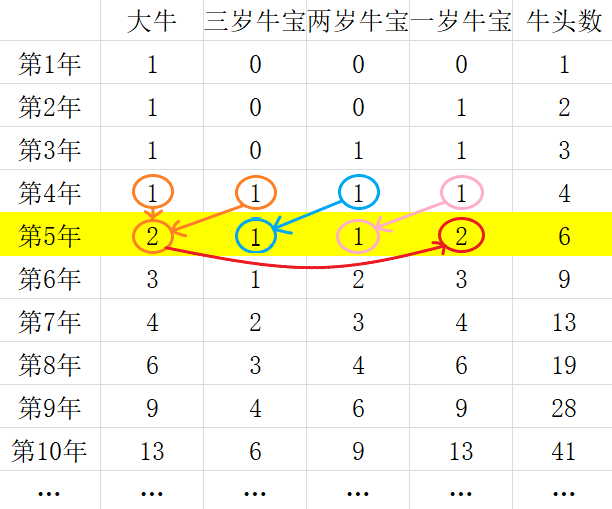#### shijilin1等　　级 排　　名 353 经　　验 4902 参赛次数 0 文章发表 1 年　　龄 99 在职情况 学生 学　　校 望子成龙小学 专　　业

自我简介：

#### TA的其他文章

 1004: [递归]母牛的故事浏览：16358

#### 你可能喜欢a[n] = a[n-1]+b[n-1]

b[n] = c[n-1]

c[n] = d[n-1]

d[n] = a[n-1]+b[n-1] = a[n]

= a[n-1]+b[n-1]+c[n-1]+d[n-1]+a[n-1]+b[n-1]

= s[n-1]+a[n-2]+b[n-2]+c[n-2]

= s[n-1]+a[n-3]+b[n-3]+c[n-3]+d[n-3]

= s[n-1]+s[n-3]

int fab(int month) {
if(month < 4) {
return month;
} else {
return fab(month-1)+fab(month-3);
}
}

#include<iostream>
using namespace std;

long long cow = {0,1,2,3,4}; // 在main函数外定义的数组会全部自动初始化为0

long long fab(int month) {
if(cow[month] == 0) { // 没有算过的月份，数组内存的是0
cow[month] = fab(month-1) + fab(month-3);
return cow[month];
} else {
return cow[month];
}
}

int main () {
int n;
while(scanf("%d", &n) && n) cout << fab(n) << endl;
return 0;
}

#include<iostream>
using namespace std;

int main () {
int cow = {0,1,2,3,4};
for(int i = 4; i < 55; i ++) cow[i] = cow[i-1] + cow[i-3];
int n;
while(scanf("%d", &n) && n) cout << cow[n] << endl;
return 0;
}

0.0分

230 人评分

#### 评论区

"应题目要求使用递归:"这个代码不对，数组cow应该是={0,1,1,1,1}
2023-05-09 22:41:19
#include<stdio.h>

int num(int n){
if(n < 5) return n;
else return num(n-1)+num(n-3);
}

int main(){
int n;
while(scanf("%d",&n) && n)
printf("%d\n",num(n));
}
returrn 0;
}

2023-01-31 19:24:13
提交了不对啊！
2023-01-26 13:17:17
果然还是得先找出递归公式啊
2022-11-10 11:18:06
真的很牛，并且帮助我理解了动态规划的思想
2022-03-09 21:25:49
很厉害
2022-02-27 20:51:58
#include<stdio.h>
int row(int x)
{
int n = 0;
if (x == 1)
return 1;
else if (x == 2)
return 2;
else if (x == 3)
return 3;
else
return row(x - 3) + row(x - 1);
}
int main()
{
int i = 0;
scanf("%d", &i);
if (i > 0&&i<55)
{
int ret = row(i);
printf("%d", ret);
}
return 0;
}

2022-01-01 09:00:31
那不就是
i=4；
a[i]=a[i-1]+a[i-3]

2021-12-29 09:14:05